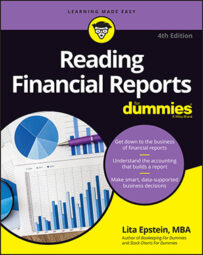##### Reading Financial Reports For DummiesLenders are always sure to look at debt on financial reports using the debt-to-capital ratio, which measures a company's leverage by looking at what portion of its capital comes from debt financing.

## How to calculate the debt-to-capital ratio

You use a three-step process to calculate the debt-to-capital ratio:

1. Find the total debt.

Total debt = Short-term borrowing + Long-term debt + Current portion of long-term debt + Notes payable

2. Find the capital.

Capital = Total debt + Equity

3. Calculate the debt-to-capital ratio.

Total debt ÷ Capital = Debt-to-capital ratio

To show you how to calculate the debt-to-capital ratio, use the information from Mattel's and Hasbro's 2007 balance sheets.

### Mattel

First, to find out Mattel's total debt, add up Mattel's short-term and long-term debt obligations:

Short-term borrowings \$9,844,000

Current portion of long-term debt \$400,000

Long-term debt \$1,100,000

Total debt \$1,509,844,000

Next, add the total debt to total equity to figure the number for capital:

\$3,067,044,000 (Equity) + \$1,509,844,000 (Debt) = \$4,576,888,000 (Capital)

Finally, calculate the debt-to-capital ratio:

\$1,509,844,000 (Total debt) ÷\$4,576,888,000 (Capital) = 0.33 (Debt-to-capital ratio)

So Mattel's debt-to-capital ratio was 0.33 to 1 in 2007.

### Hasbro

First, to find out Hasbro's total debt, add up Hasbro's short-term and long-term debt obligations:

Short-term borrowings \$224,365,000

Current portion of long-term debt \$0

Long-term debt \$1,396,421,000

Total debt \$1,620,786,000

Then add the total debt to total equity to find out the number for capital:

\$1,507,379,000 (Equity) + \$1,620,786,000 (Debt) = \$3,128,165,000 (Capital)

Finally, calculate the debt-to-capital ratio:

\$1,620,786,000 (Total debt) ÷ \$3,128,165,000 (Capital) = 0.52 (Debt-to-capital ratio)

So Hasbro's debt-to-capital ratio was higher than Mattel's, at 0.52 to 1.

## What do the numbers mean?

Lenders often place debt-to-capital ratio requirements in the terms of a credit agreement for a company to maintain its credit status. If a company's debt creeps above what its lenders allow for the debt-to-capital ratio, the lender can call the loan, which means the business has to raise cash to pay off the loan.

Companies usually take care of a call by finding another lender. The new lender likely charges higher interest rates because the company's higher debt-to-capital ratio makes the company appear as though it's a greater credit risk.

Generally, companies are considered to be in good financial shape with a debt-to-capital ratio of 0.35 to 1 or less. If a company's debt-to-capital ratio creeps above 0.50 to 1, lenders usually consider the company a much higher credit risk, which means it has to pay higher interest rates to get loans.

Take note of the ratio and how it compares with the ratios of similar companies in its industry. If the company has a higher debt-to-capital ratio than most of its competitors, lenders probably see it as a much higher credit risk.

A company with a higher-than-normal debt-to-capital ratio faces an increasing cost of operating as it tries to meet the obligations of paying higher interest rates. These higher interest payments can spiral into more significant problems as the cash crunch intensifies.

In a worst-case scenario, the company can seek bankruptcy protection from its creditors to continue operating and to restructure its debt. Many times its stock value plummets — and may have no value at all if the company emerges from bankruptcy.